The GLM Procedure

### Homogeneity of Variance in One-Way Models

One of the usual assumptions in using the GLM procedure is that the underlying errors are all uncorrelated with homogeneous variances. You can test this assumption in PROC GLM by using the HOVTEST option in the MEANS statement, requesting a homogeneity of variance test. This section discusses the computational details behind these tests. Note that the GLM procedure allows homogeneity of variance testing for simple one-way models only. Homogeneity of variance testing for more complex models is a subject of current research.

Bartlett (1937) proposes a test for equal variances that is a modification of the normal-theory likelihood ratio test (the HOVTEST=BARTLETT option). While Bartlett’s test has accurate Type I error rates and optimal power when the underlying distribution of the data is normal, it can be very inaccurate if that distribution is even slightly nonnormal (Box; 1953). Therefore, Bartlett’s test is not recommended for routine use.

An approach that leads to tests that are much more robust to the underlying distribution is to transform the original values of the dependent variable to derive a dispersion variable and then to perform analysis of variance on this variable. The significance level for the test of homogeneity of variance is the p-value for the ANOVAtest on the dispersion variable. All of the homogeneity of variance tests available in PROC GLM except Bartlett’s use this approach.

Levene’s test (Levene; 1960) is widely considered to be the standard homogeneity of variance test (the HOVTEST=LEVENE option). Levene’s test is of the dispersion-variable-ANOVA form discussed previously, where the dispersion variable is either of the following: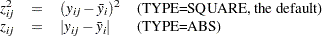O’Brien (1979) proposes a test (HOVTEST=OBRIEN) that is basically a modification of Levene’s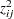, using the dispersion variable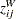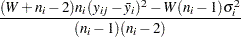where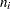is the size of theth group and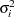is its sample variance. You can use the W= option in parentheses to tune O’Brien’s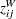dispersion variable to match the suspected kurtosis of the underlying distribution. The choice of the value of the W= option is rarely critical. By default, W=0.5, as suggested by O’Brien (1979, 1981).

Finally, Brown and Forsythe (1974) suggest using the absolute deviations from the group medians: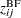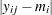whereis the median of theth group. You can use the HOVTEST=BF option to specify this test.

Simulation results (Conover, Johnson, and Johnson 1981; Olejnik and Algina 1987) show that, while all of these ANOVA-based tests are reasonably robust to the underlying distribution, the Brown-Forsythe test seems best at providing power to detect variance differences while protecting the Type I error probability. However, since the within-group medians are required for the Brown-Forsythe test, it can be resource intensive if there are very many groups or if some groups are very large.

If one of these tests rejects the assumption of homogeneity of variance, you should use Welch’s ANOVA instead of the usual ANOVA to test for differences between group means. However, this conclusion holds only if you use one of the robust homogeneity of variance tests (that is, not for HOVTEST=BARTLETT); even then, any homogeneity of variance test has too little power to be relied upon to always detect when Welch’s ANOVA is appropriate. Unless the group variances are extremely different or the number of groups is large, the usual ANOVA test is relatively robust when the groups are all about the same size. As Box (1953) notes, "To make the preliminary test on variances is rather like putting to sea in a rowing boat to find out whether conditions are sufficiently calm for an ocean liner to leave port!"

Example 39.10 illustrates the use of the HOVTEST and WELCH options in the MEANS statement in testing for equal group variances and adjusting for unequal group variances in a one-way ANOVA.Previous Page | Next Page | Top of Page